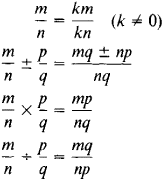rational number

Also found in: Dictionary, Thesaurus, Medical, Legal, Financial, Acronyms, Wikipedia.
Related to rational number: real number, irrational number

rational number:

see numbernumber,
entity describing the magnitude or position of a mathematical object or extensions of these concepts. The Natural Numbers

Cardinal numbers describe the size of a collection of objects; two such collections have the same (cardinal) number of objects if their
.
The following article is from The Great Soviet Encyclopedia (1979). It might be outdated or ideologically biased.

Rational Number

a number that can be expressed in the form of a fraction m/n, where m and η are integers and n ≠ 0. Since an integer m can be expressed as m/1, all integers are rational numbers. The operations of addition, subtraction, multiplication, and division (by nonzero divisors) can always be performed in the domain of rational numbers; thus, the rational numbers form a field. The basic rules of the operations over the rational numbers are given by the formulasRational numbers can also be represented in the form of finite decimals or infinite periodic decimals. Every irrational number can be included between two rational numbers, the difference between which can be made arbitrarily small.

rational number

[′rash·ən·əl ′nəm·bər]
(mathematics)
A number which is the quotient of two integers.
McGraw-Hill Dictionary of Scientific & Technical Terms, 6E, Copyright © 2003 by The McGraw-Hill Companies, Inc.

rational number

any real number of the form a/b, where a and b are integers and b is not zero, as 7 or 7/3
Collins Discovery Encyclopedia, 1st edition © HarperCollins Publishers 2005
References in periodicals archive ?
The other text was Making Sense of Fractions, Ratios, and Proportions (NCTM 2002), the 64th yearbook from the National Council of Teachers of Mathematics (NCTM) that focused specifically on recent research related to issues with rational numbers and offered us a wider view of the existing research.
St.: Hence [square root of 2] is not a rational number; but I know it to have a decimal expansion ...
From fractions to rational numbers of arithmetic: A reorganization hypothesis.
Students have difficulty translating one rational number model, whether verbal, pictorial, or symbolic, into another.
A rational number a/b is represented by a finite continued fraction, that is, a number of the form
[S.sub.a,n]+ and [S.sub.b,m]+, R[[S.sub.b]+, [S.sub.a]+] is at least as great as any rational number in
Whole numbers and fractions together make up the rational numbers (those that can be expressed as ratios), and it is easy to suppose that rational numbers are all that exist.
Since integers are closed for addition and multiplication, AD= an integer BC= an integer BD= an integer AD + BC = an integer + an integer = another integer Therefore, (AD + BC)/BD = integer/ integer and by definition, a rational number is the ratio of two integers, so the proof is complete.
The Galois group of f (a, x) is isomorphic to [C.sub.4] [??] [C.sub.2] if and only if a [not equal to] [n.sup.2] - 1/2n with a rational number n.
Kyril Dombrowski  mentioned that oscillating systems --having the peculiarity to change their own parameters because of interactions inside the systems--have a tendency to reach a stable state where the individual oscillator frequencies are interrelated by specific numbers--namely minima of the rational number density on the number line.
But in my experience, many calculators resort to rational number finite-decimal approximations, further truncated by the limited number of significant figures used or displayed by the calculator.
Intermediate teachers' knowledge of rational number concepts.

Site: Follow: Share:
Open / Close